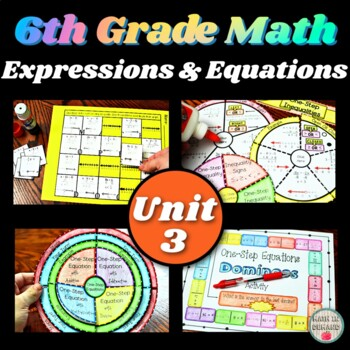### 6th Grade Math Unit 3 Expressions & Equations Curriculum

This bundle includes all of my 6th Grade Math Unit 3 Resources (6.EE - Expressions & Equations). There are interactive notebook pages, activities, foldables, practice worksheets, homework, exit slips, assessments (pre- and post-tests), vocabulary quizzes, warm-ups, and more! There are also some digital resources such as early finishers.All of the pre-tests and post-tests are editable. The early finishers are given in PowerPoint. You can upload the file into Google Slides and share the link with students.Math concepts included are:

1.) Intro to Variables

2.) Evaluating Expressions

3.) Writing Algebraic Expressions

4.) Solving One-Step Equations

5.) Intro to Inequalities

6.) Solving One-Step Inequalities

7.) Dependent vs Independent Variables

8.) Combining Like Terms

9.) The Distributive Property

10.) Writing Equivalent Expressions

Check out the PREVIEW to see more!!!I also have other units for 6th Grade Math

Unit 1 - Number Sense

Unit 2 - Ratios & Proportional Relationships

Unit 4 - Geometry

Unit 5 - Statistics & Probability